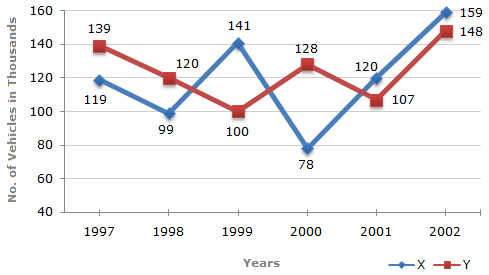# Data Interpretation - Line Charts - Discussion

### Discussion :: Line Charts - Line Chart 3 (Q.No.4)

Study the following line graph and answer the questions based on it.

Number of Vehicles Manufactured by Two companies ove the Years (Number in Thousands)4.

In which of the following years, the difference between the productions of Companies X and Y was the maximum among the given years ?

 [A]. 1997 [B]. 1998 [C]. 1999 [D]. 2000

Explanation:

The difference between the productions of Companies X and Y in various years are:

For 1997 (139000 - 119000) = 20000.

For 1998 (120000 - 99000) = 21000.

For 1999 (141000 - 100000) = 41000.

For 2000 (128000 - 78000) = 50000.

For 2001 (120000 - 107000) = 13000.

For 2002 (159000 - 148000) = 11000.

Clearly, maximum difference was in 2000.

 Ankit said: (Mar 5, 2015) This answer is wrong as sign should be considered here question is difference between x and y means x-y not y-x.

 Sandeep said: (Jun 26, 2015) Question asked only highest difference.

 Sam said: (Apr 6, 2017) It should be year 2002 as the difference is minimum in year 2002.